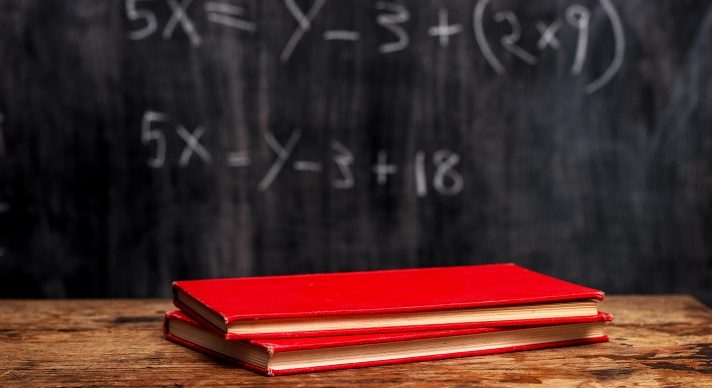# Integrated Mathematics I### Course Overview

Acellus Integrated Mathematics I, taught by Patrick Mara, is the first course of a three-part series that includes algebra, geometry, probability, and statistics. This high school math pathway is patterned after an approach typically seen internationally. Acellus Integrated Mathematics I is A-G Approved through the University of California.This course was developed by the International Academy of Science. Learn More

### Scope and Sequence

Unit 1 This introductory unit of this course introduces variables and expressions, order of operations, real numbers, properties, adding & subtracting, and multiplying & dividing. This unit also includes the distributive property, introduction to equations, and two variable equations. Unit 2 This unit discusses one step equations, two step equations, multi-step equations, variables on both sides, literal equations, ratios and rates, conversions, similar figures, as well as percents, and percent change. Unit 3 Within this unit students will learn about inequalities, addition & subtraction with inequalities, multiplication & division with inequalities, multi-step inequalities, sets, and interval notation. Students will also learn about compound inequalities, absolute value equations, and absolute value inequalities. Unit 4 In this unit, students learn about two variable graphs, patterns, non-linear graphs, graphing a function, writing a rule, and relations & functions. The unit also introduces the composition of functions and arithmetic sequences. Unit 5 This unit guides students through rate of change, direct variation, slope-intercept, point-slope, standard form, parallel & perpendicular, as well as scatter plots & trend lines, and absolute value functions.

Following this unit students are presented with the Mid-term Review and Exam.

Unit 6 Students will gain the knowledge of solving systems by graphing, substitution, elimination, and applications of systems. Students will also be introduced to linear inequalities and systems of inequalities. Unit 7 This unit discusses zero & negative exponents, multiplying powers, more multiplication properties, division properties, rational exponents, exponential functions , as well as exponential growth & decay, geometric sequences, and mathematical modeling. Unit 8 Students begin this unit by learning about frequency & histograms, statistical measures, as well as the usefulness and importance of  box and whisker plots and two-way tables. Unit 9 In this unit students will learn nets and perspective drawings, points, lines, and planes, measuring segments, measuring angles, angle pairs, and basic constructions. This unit also introduces coordinate planes and perimeter, circumference, and area. Unit 10 Within this unit students gain knowledge concerning transversals, parallel lines, proving parallel lines, and parallel and perpendicular lines. Students also be introduces to angle and theorem, more constructions, equations of lines, and slopes of parallel and perpendicular lines. Unit 11 This unit discusses translations, reflections, rotations, compositions, congruence transformations, as well as dilations, and similarity transformations. Unit 12 In the concluding unit of this course, students will learn about areas of parallelograms and triangles, areas of trapezoids, rhombuses, and kites, areas of regular polygons, circles and arcs, and lastly the length of arcs.

Following this unit students are presented with the Final Review and Exam.